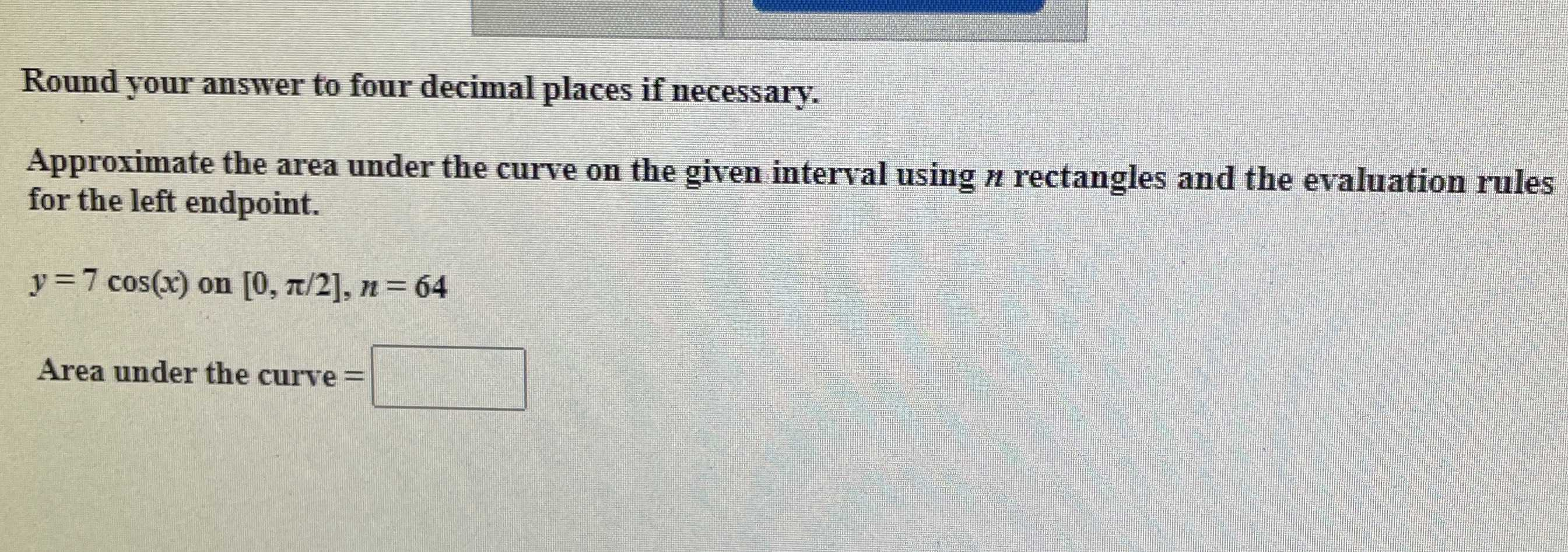### Still have math questions?

Trigonometry
QuestionApproximate the area under the curve on the given interval using $$n$$ rectangles and the evaluation rules for the left endpoint.
$$y = 7 \cos ( x )$$ on $$[ 0 , \pi / 2 ] , n = 64$$